# 25 Results

View
Selected filters:
• CCSS.Math.Content.8.G.B.7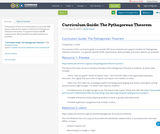Conditional Remix & Share Permitted
CC BY-NC
Rating

The purpose of this curriculum guide is to provide OER lesson material and support activities for Pythagorean Theorem instruction. It is geared towards GED® requirements. Both printable and online options are provided.

Subject:
Mathematics
Material Type:
Activity/Lab
Assessment
Lesson
Teaching/Learning Strategy
Author:
Shana Friend
05/30/2018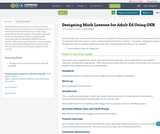Conditional Remix & Share Permitted
CC BY-NC-SA
Rating

Adult Educators do not have a lot of time to dive into dozens of resources spread all over the internet to try to find the essential items they need to create a high-quality lesson for their students. This guide is designed to walk you through each step of creating a math lesson-plan with recommended OER that you can evaluate.

With each step of the guide, I create a sample lesson on learning the Pythagorean Theorem. By the end, you have an entire lesson plan example for this concept.

Subject:
Mathematics
Material Type:
Activity/Lab
Lesson Plan
Teaching/Learning Strategy
Author:
Jason Walker
05/18/2018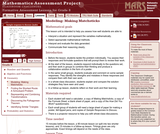Only Sharing Permitted
CC BY-NC-ND
Rating

This lesson unit is intended to help you assess how well students are able to: interpret a situation and represent the variables mathematically; select appropriate mathematical methods; interpret and evaluate the data generated; and communicate their reasoning clearly.

Subject:
Mathematics
Material Type:
Assessment
Lesson Plan
Provider:
Shell Center for Mathematical Education
U.C. Berkeley
Provider Set:
Mathematics Assessment Project (MAP)
04/26/2013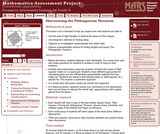Only Sharing Permitted
CC BY-NC-ND
Rating

This lesson unit is intended to help teachers assess how well students are able to: use the area of right triangles to deduce the areas of other shapes; use dissection methods for finding areas; organize an investigation systematically and collect data; deduce a generalizable method for finding lengths and areas (The Pythagorean Theorem.)

Subject:
Geometry
Material Type:
Assessment
Lesson Plan
Provider:
Shell Center for Mathematical Education
U.C. Berkeley
Provider Set:
Mathematics Assessment Project (MAP)
04/26/2013Only Sharing Permitted
CC BY-NC-ND
Rating

This lesson unit is intended to help you assess how students reason about geometry and, in particular, how well they are able to: use facts about the angle sum and exterior angles of triangles to calculate missing angles; apply angle theorems to parallel lines cut by a transversal; interpret geometrical diagrams using mathematical properties to identify similarity of triangles.

Subject:
Geometry
Material Type:
Assessment
Lesson Plan
Provider:
Shell Center for Mathematical Education
U.C. Berkeley
Provider Set:
Mathematics Assessment Project (MAP)
04/26/2013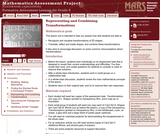Only Sharing Permitted
CC BY-NC-ND
Rating

This lesson unit is intended to help teachers assess how well students are able to: recognize and visualize transformations of 2D shapes; and translate, reflect and rotate shapes, and combine these transformations. It also aims to encourage discussion on some common misconceptions about transformations.

Subject:
Geometry
Material Type:
Assessment
Lesson Plan
Provider:
Shell Center for Mathematical Education
U.C. Berkeley
Provider Set:
Mathematics Assessment Project (MAP)
04/26/2013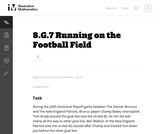Unrestricted Use
CC BY
Rating

This is a task from the Illustrative Mathematics website that is one part of a complete illustration of the standard to which it is aligned. Each task has at least one solution and some commentary that addresses important asects of the task and its potential use. Here are the first few lines of the commentary for this task: During the 2005 Divisional Playoff game between The Denver Broncos and The New England Patriots, Bronco player Champ Bailey intercepted Tom Brady aroun...

Subject:
Mathematics
Material Type:
Activity/Lab
Provider:
Illustrative Mathematics
Provider Set:
Illustrative Mathematics
Author:
Illustrative Mathematics
03/17/2013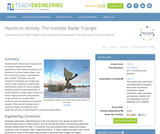Educational Use
Rating

Students learn about radar imaging and its various military and civilian applications that include recognition and detection of human-made targets, and the monitoring of space, deforestation and oil spills. They learn how the concepts of similarity and scaling are used in radar imaging to create three-dimensional models of various targets. Students apply the critical attributes of similar figures to create scale models of a radar imaging scenario using infrared range sensors (to emulate radar functions) and toy airplanes (to emulate targets). They use technology tools to measure angles and distances, and relate the concept of similar figures to real-world applications.

Subject:
Education
Material Type:
Activity/Lab
Provider:
TeachEngineering
Provider Set:
TeachEngineering
Author:
Mounir Ben Ghalia
Rocio Denise Nava
10/14/2015
Remix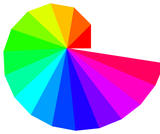Unrestricted Use
CC BY
Rating

This is a cross curricular art project for 8th grade math students. Students are first introduced to what the Wheel of Theodorus is, ponder where they see it in the world around them and then instructed on how to create their own. When they have finished constructing their Wheel of Theodorus they are asked to creatively and colorfully turn it "into" something. Examples are given. After they Wheel of Theodorus is complete, students are then asked to measure all the sides lengths of the triangles in the wheel. They should quickly see that they can use the Pythagorean Theorem to do this and that it follows a predictable pattern. No ruler will be required for this part of the project!

Subject:
Mathematics
Geometry
Material Type:
Activity/Lab
Homework/Assignment
Author:
Jennifer Chandler
Sara Scholes
03/26/2020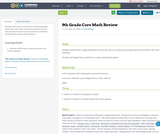Conditional Remix & Share Permitted
CC BY-NC
Rating

Students will create an interactive Powerpoint game where they will create real world problems that are used as clues to move on to the next level. Problems include all 8th grade math standards.

Subject:
Mathematics
Material Type:
Interactive
Author:
Linda Ridings
01/28/2016Educational Use
Rating

Students learn that math is important in navigation and engineering. They learn about triangles and how they can help determine distances. Ancient land and sea navigators started with the most basic of navigation equations (speed x time = distance). Today, navigational satellites use equations that take into account the relative effects of space and time. However, even these high-tech wonders cannot be built without pure and simple math concepts â basic geometry and trigonometry â that have been used for thousands of years.

Subject:
Education
Material Type:
Activity/Lab
Provider:
TeachEngineering
Provider Set:
TeachEngineering
Author:
Janet Yowell
Jeff White
Malinda Schaefer Zarske
Matt Lippis
10/14/2015Unrestricted Use
CC BY
Rating

This is a cross curricular art project for 8th grade math students. Students are first introduced to what the Wheel of Theodorus is, ponder where they see it in the world around them and then instructed on how to create their own. When they have finished constructing their Wheel of Theodorus they are asked to creatively and colorfully turn it "into" something. Examples are given. After they Wheel of Theodorus is complete, students are then asked to measure all the sides lengths of the triangles in the wheel. They should quickly see that they can use the Pythagorean Theorem to do this and that it follows a predictable pattern. No ruler will be required for this part of the project!

Subject:
Mathematics
Geometry
Material Type:
Lesson Plan
Author:
Sara Scholes
10/06/2017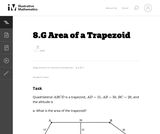Unrestricted Use
CC BY
Rating

This is a task from the Illustrative Mathematics website that is one part of a complete illustration of the standard to which it is aligned. Each task has at least one solution and some commentary that addresses important asects of the task and its potential use. Here are the first few lines of the commentary for this task: Quadrilateral $ABCD$ is a trapezoid, $AD = 15$, $AB = 50$, $BC = 20$, and the altitude is 12. What is the area of the trapezoid?...

Subject:
Mathematics
Material Type:
Activity/Lab
Provider:
Illustrative Mathematics
Provider Set:
Illustrative Mathematics
Author:
Illustrative Mathematics
03/17/2013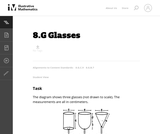Unrestricted Use
CC BY
Rating

This task gives students an opportunity to work with volumes of cylinders, spheres and cones. Notice that the insight required increases as you move across the three glasses, from a simple application of the formula for the volume of a cylinder, to a situation requiring decomposition of the volume into two pieces, to one where a height must be calculated using the Pythagorean theorem.

Subject:
Mathematics
Geometry
Material Type:
Activity/Lab
Provider:
Illustrative Mathematics
Provider Set:
Illustrative Mathematics
Author:
Illustrative Mathematics
05/01/2012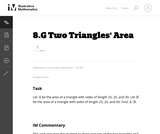Unrestricted Use
CC BY
Rating

This is a task from the Illustrative Mathematics website that is one part of a complete illustration of the standard to which it is aligned. Each task has at least one solution and some commentary that addresses important asects of the task and its potential use. Here are the first few lines of the commentary for this task: Let $A$ be the area of a triangle with sides of length 25, 25, and 30. Let $B$ be the area of a triangle with sides of length 25, 25, and 40. Find A/B... Subject: Mathematics Material Type: Activity/Lab Provider: Illustrative Mathematics Provider Set: Illustrative Mathematics Author: Illustrative Mathematics Date Added: 05/29/2013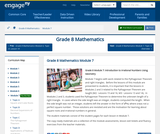Conditional Remix & Share Permitted CC BY-NC-SA Rating Module 7 begins with work related to the Pythagorean Theorem and right triangles. Before the lessons of this module are presented to students, it is important that the lessons in Modules 2 and 3 related to the Pythagorean Theorem are taught (M2: Lessons 15 and 16, M3: Lessons 13 and 14). In Modules 2 and 3, students used the Pythagorean Theorem to determine the unknown length of a right triangle. In cases where the side length was an integer, students computed the length. When the side length was not an integer, students left the answer in the form of x2=c, where c was not a perfect square number. Those solutions are revisited and are the motivation for learning about square roots and irrational numbers in general. Subject: Geometry Material Type: Module Provider: New York State Education Department Provider Set: EngageNY Date Added: 02/02/2014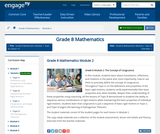Conditional Remix & Share Permitted CC BY-NC-SA Rating In this module, students learn about translations, reflections, and rotations in the plane and, more importantly, how to use them to precisely define the concept of congruence. Throughout Topic A, on the definitions and properties of the basic rigid motions, students verify experimentally their basic properties and, when feasible, deepen their understanding of these properties using reasoning. All the lessons of Topic B demonstrate to students the ability to sequence various combinations of rigid motions while maintaining the basic properties of individual rigid motions. Students learn that congruence is just a sequence of basic rigid motions in Topic C, and Topic D begins the learning of Pythagorean Theorem. Subject: Geometry Material Type: Module Provider: New York State Education Department Provider Set: EngageNY Date Added: 09/21/2013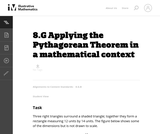Unrestricted Use CC BY Rating This task requires students to apply the Pythagorean Theorem. Subject: Mathematics Geometry Material Type: Activity/Lab Provider: Illustrative Mathematics Provider Set: Illustrative Mathematics Author: Illustrative Mathematics Date Added: 05/01/2012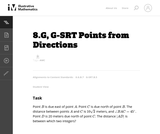Unrestricted Use CC BY Rating This is a task from the Illustrative Mathematics website that is one part of a complete illustration of the standard to which it is aligned. Each task has at least one solution and some commentary that addresses important asects of the task and its potential use. Here are the first few lines of the commentary for this task: PointB$is due east of point$A$. Point$C$is due north of point$B$. The distance between points$A$and$C$is$10\sqrt 2$meters, and$\angle BAC...

Subject:
Mathematics
Material Type:
Activity/Lab
Provider:
Illustrative Mathematics
Provider Set:
Illustrative Mathematics
Author:
Illustrative Mathematics
03/29/2013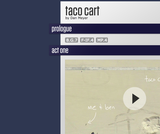Conditional Remix & Share Permitted
CC BY-NC
Rating

The 3 act math is a perfect way to incorporate the See-Think-Wonder activity.  Dan Meyer is the originator of the 3 act math plays and the Pearson Envisions Math textbook incorporates one every Topic/Chapter.  I am writing this lesson plan with the idea of using the Taco Cart 3 act math play.The link to the 3-act-math play is below.Taco Cart http://threeacts.mrmeyer.com/tacocart/

Subject:
Mathematics
Material Type:
Lesson Plan
Author:
Jill Oliver